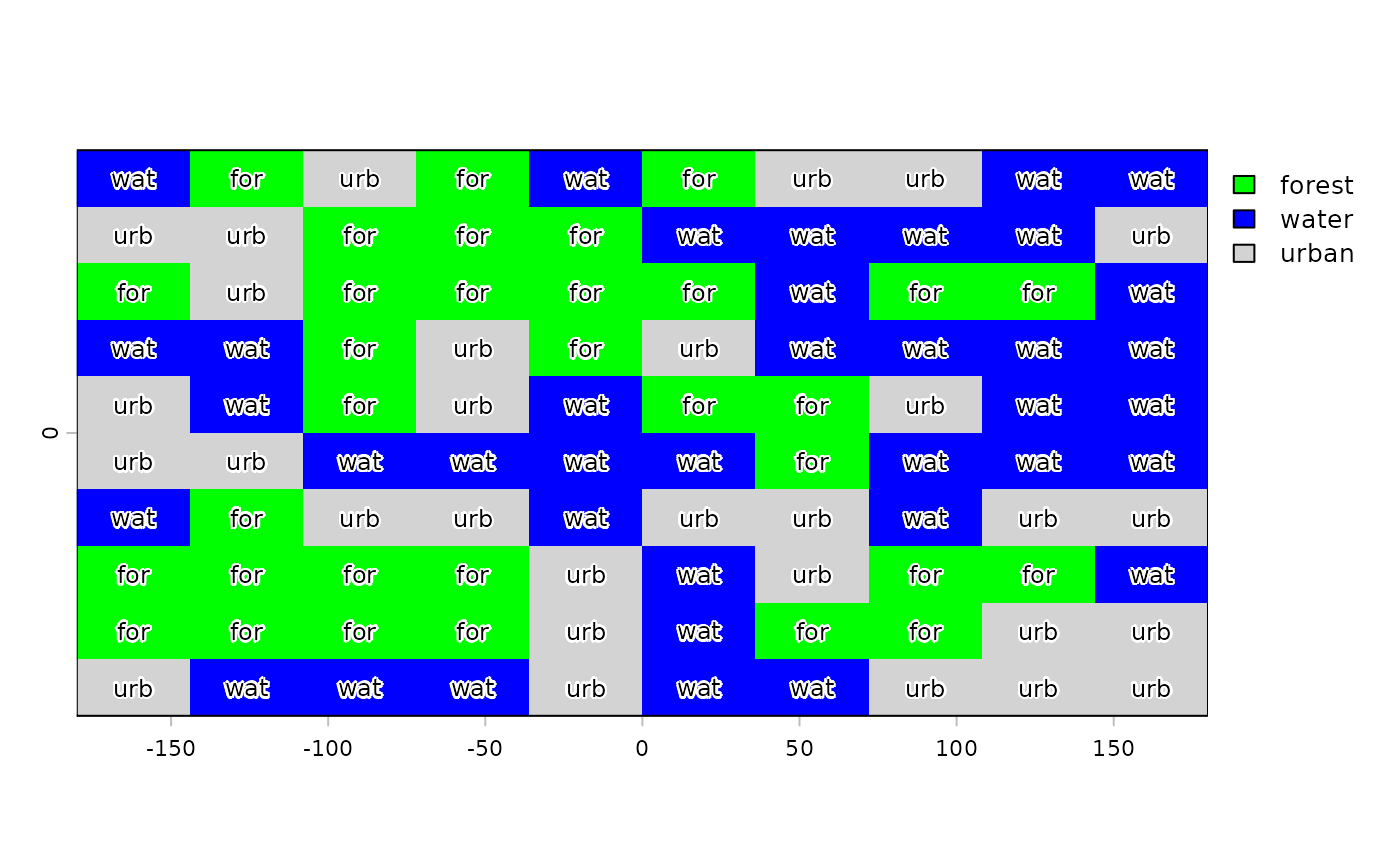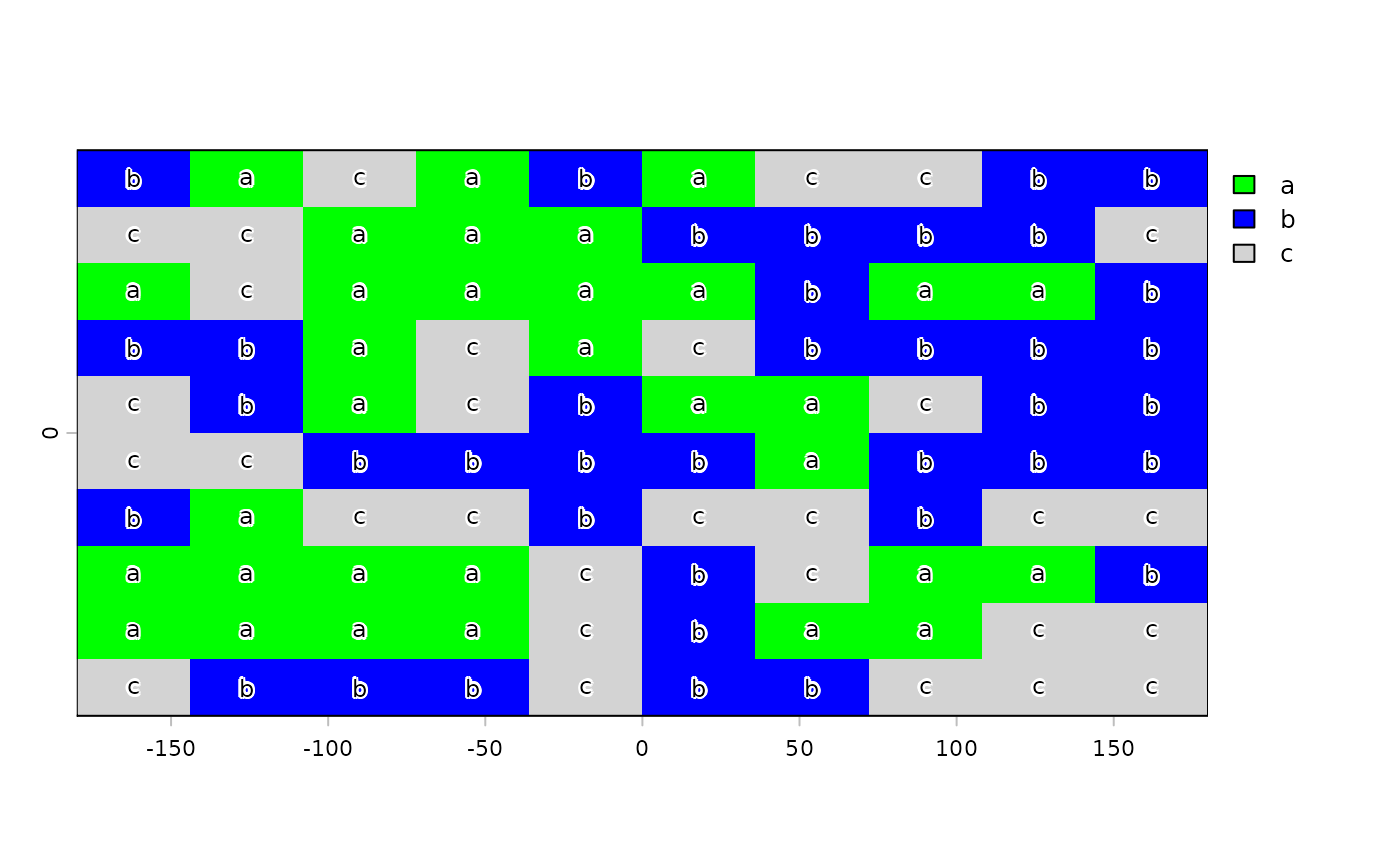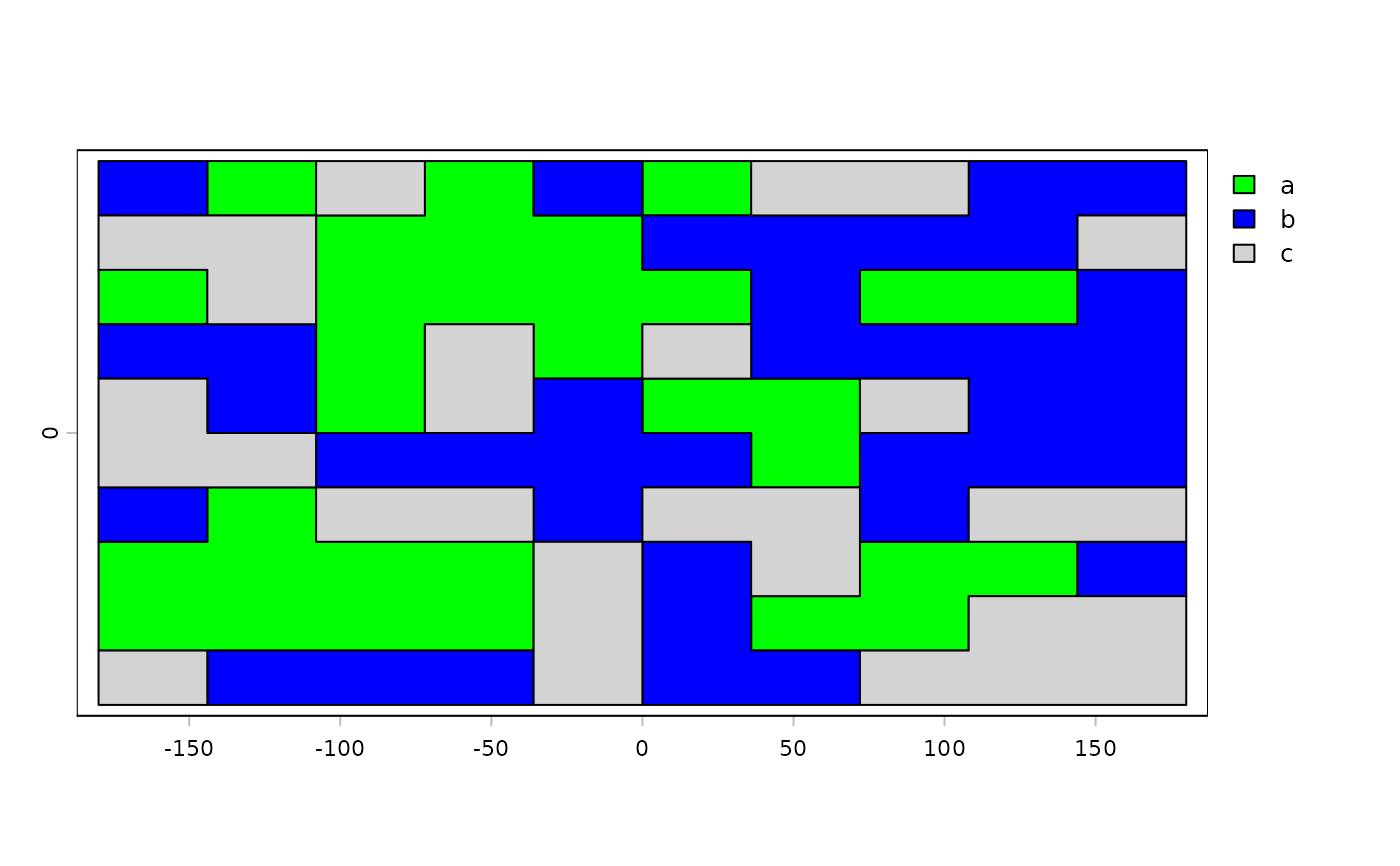A SpatRaster layer can represent a categorical variable (factor). Like factors, SpatRaster categories are stored as integers that have an associated label.

The categories can be inspected with levels and cats. They are represented by a data.frame that must have two or more columns, the first one identifying the (integer) cell values and the other column(s) providing the category labels.

If there are multiple columns with categories, you can set the "active" category to choose the one you want to use.

cats returns the entire data.frame, whereas levels only return two columns: the index and the active category.

To set categories for the first layer of a SpatRaster, you can provide levels<- with a data.frame or a list with a data.frame. To set categories for multiple layers you can provide levels<- with a list with one element (that either has a data.frame or is NULL) for each layer. Use categories to set the categories for a specific layer or specific layers.

droplevels removes categories that are not used (declared but not present as values in the raster) if levels=NULL.

addCats adds additional categories to a layer that already is categorical. It adds new variables, not new levels of an existing categorical variable.

# S4 method for SpatRaster
levels(x)

# S4 method for SpatRaster
levels(x)<-value

# S4 method for SpatRaster
cats(x, layer)

# S4 method for SpatRaster
categories(x, layer=1, value, active=1, ...)

# S4 method for SpatRaster
droplevels(x, level=NULL, layer=1)

# S4 method for SpatRaster
addCats(x, value, merge=FALSE, layer=1)

## Arguments

x

SpatRaster

layer

the layer name or number (positive integer); or 0 for all layers

value

a data.frame (ID, category) that define the categories. Or NULL to remove them

active

positive integer, indicating the column in value to be used as the active category (zero based to skip the first column with the cell values; that is 1 is the second column in value)

level

the categories to remove for the layer specified with layer

merge

logical. If TRUE, the categories are combined with merge using the first column of value as ID. If FALSE the categories are combined with cbind

...

## Value

list of data.frames (levels, cats) or logical (is.factor)

activeCat, catalyze, set.cats, as.factor, is.factor

## Examples

set.seed(0)
r <- rast(nrows=10, ncols=10)
values(r) <- sample(3, ncell(r), replace=TRUE)
is.factor(r)
#>  FALSE

cls <- data.frame(id=1:3, cover=c("forest", "water", "urban"))
levels(r) <- cls
is.factor(r)
#>  TRUE
r
#> class       : SpatRaster
#> dimensions  : 10, 10, 1  (nrow, ncol, nlyr)
#> resolution  : 36, 18  (x, y)
#> extent      : -180, 180, -90, 90  (xmin, xmax, ymin, ymax)
#> coord. ref. : lon/lat WGS 84
#> source(s)   : memory
#> categories  : cover
#> name        :  cover
#> min value   : forest
#> max value   :  urban

plot(r, col=c("green", "blue", "light gray"))
text(r, digits=3, cex=.75, halo=TRUE)# raster starts at 3
x <- r + 2
is.factor(x)
#>  FALSE

# Multiple categories
d <- data.frame(id=3:5, cover=cls[,2], letters=letters[1:3], value=10:12)
levels(x) <- d
x
#> class       : SpatRaster
#> dimensions  : 10, 10, 1  (nrow, ncol, nlyr)
#> resolution  : 36, 18  (x, y)
#> extent      : -180, 180, -90, 90  (xmin, xmax, ymin, ymax)
#> coord. ref. : lon/lat WGS 84
#> source(s)   : memory
#> categories  : cover, letters, value
#> name        :  cover
#> min value   : forest
#> max value   :  urban

# get current index
activeCat(x)
#>  1
# set index
activeCat(x) <- 3
activeCat(x)
#>  3
activeCat(x) <- "letters"
plot(x, col=c("green", "blue", "light gray"))
text(x, digits=3, cex=.75, halo=TRUE)r <- as.numeric(x)
r
#> class       : SpatRaster
#> dimensions  : 10, 10, 1  (nrow, ncol, nlyr)
#> resolution  : 36, 18  (x, y)
#> extent      : -180, 180, -90, 90  (xmin, xmax, ymin, ymax)
#> coord. ref. : lon/lat WGS 84
#> source(s)   : memory
#> name        : cover
#> min value   :     1
#> max value   :     3

p <- as.polygons(x)
plot(p, "letters", col=c("green", "blue", "light gray"))